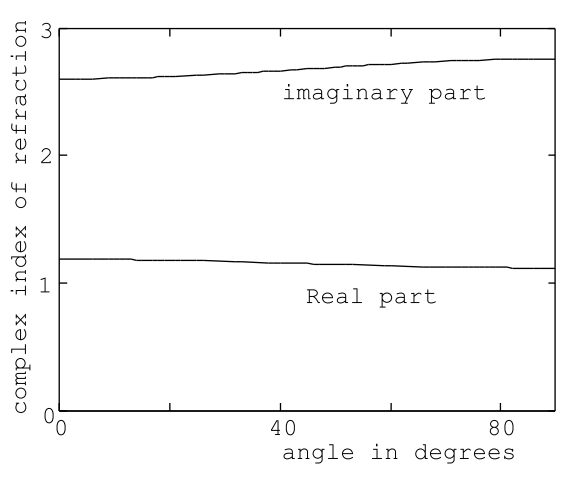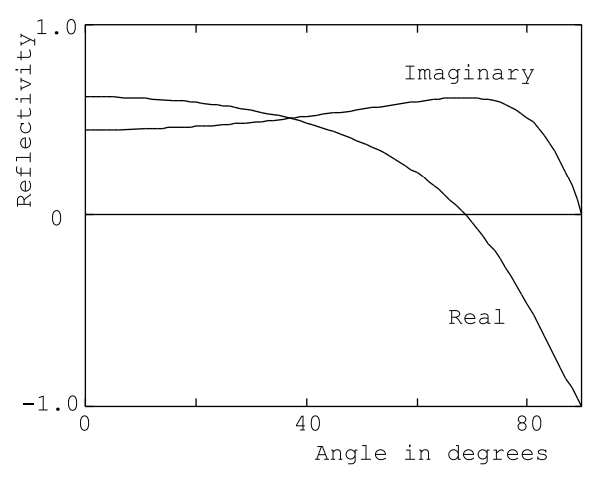Skip to main content
$$\require{cancel}$$

# 10.6: Example- Copper

The real and imaginary parts $$\left(\mathrm{n}_{\theta}+i \kappa_{\theta}\right)=\sqrt{\epsilon_{\mathrm{r}}-\sin ^{2} \theta}$$ have been plotted in Figure (10.6.8) as a function of the angle of incidence, θ, for room temperature copper and for a wavelength of λ= 0.5145 microns (see Table(10.1)). As can be seen from the figure, the angular dependence of the indices nθ, $$\kappa$$θ is not very pronounced. For a lossy material such as copper that has a complex dielectric constant the reflectivity, ER/E0, is complex; that is, the phase shift between the incident wave and reflected wave electric vectors is neither 0 (in phase) nor 180 (out of phase). The real and imaginary parts of the reflectivity have been plotted in Figure (10.6.9) as a function of the angle of incidence for S-polarized 0.5145 micron light incident on room temperature copper; the absolute value of the reflectivity has been plotted in Figure (10.6.10).Figure $$\PageIndex{8}$$: The dependence of the complex index of refraction, nθ + i$$\kappa$$θ, upon angle of incidence of the incident wave calculated for copper at room temperature and for an incident wavelength of λ = 0.5145 µm. The normal component of the wave-vector in copper is given by $$k_{z}=\left(\frac{\omega}{c}\right)\left(n_{\theta}+i \kappa_{\theta}\right)$$. At this wavelength the relative dielectric constant for copper is $$\epsilon_{r}$$=(-5.34+i6.19), n=1.19, and $$\kappa$$=2.60.Figure $$\PageIndex{9}$$: The real and imaginary part of the reflectivity of copper, ER/E0, as a function of angle of incidence for a wavelength λ= 0.5145 µm and S-polarized radiation. Copper at room temperature; $$\epsilon_{r}$$= (-5.34 + i6.19), n=1.19, and $$\kappa$$=2.60.

Similarly, the real and the imaginary parts of the ratio HR/H0 have been plotted in Figure (10.6.11) as a function of the angle of incidence for P-polarized 0.5145 micron light incident on copper; the absolute value of this ratio is shown in Figure (10.6.12). The reflection coefficient for P-polarized radiation is given by RP = ER/E0 but this is very closely related to the ratio HR/H0 because E0 = Z0H0 and ER = −Z0HR, where Z0= 377 Ohms, the impedance of free space. Notice that the real part of the reflectivity for P-polarized light vanishes at an angle of incidence of approximately 69 ; the phase of the reflected light at that angle is shifted by 90 relative to the incident light. The phase shift between reflected and incident light is much less pronounced for S-polarized light; approximately 15 for an angle of incidence of 69 .Figure $$\PageIndex{10}$$: The absolute value of the reflectivity of copper, | ER/E0 |, as a function of angle of incidence for a wavelength λ= 0.5145 µm and S-polarized radiation. Copper at room temperature; $$\epsilon_{r}$$=(-5.34 + i6.19), n=1.19, and $$\kappa$$=2.60.Figure $$\PageIndex{11}$$: The real and imaginary parts of the complex ratio HR/H0 for copper as a function of angle of incidence for a wavelength λ= 0.5145 µm and for P-polarized radiation. Copper at room temperature; $$\epsilon_{r}$$=(-5.34 + i6.19), n=1.19, and $$\kappa$$=2.60.
• Was this article helpful?